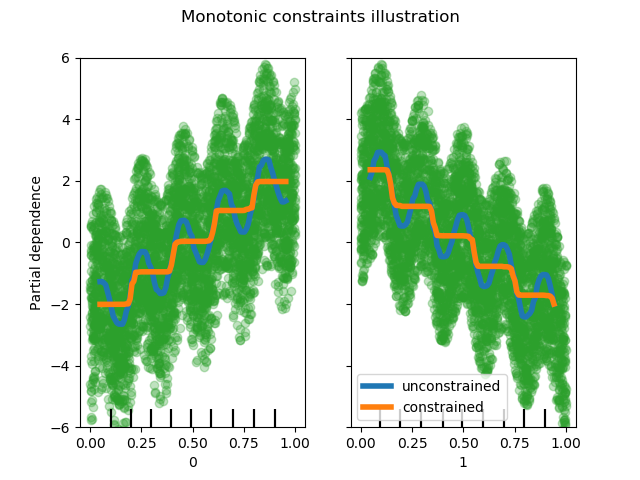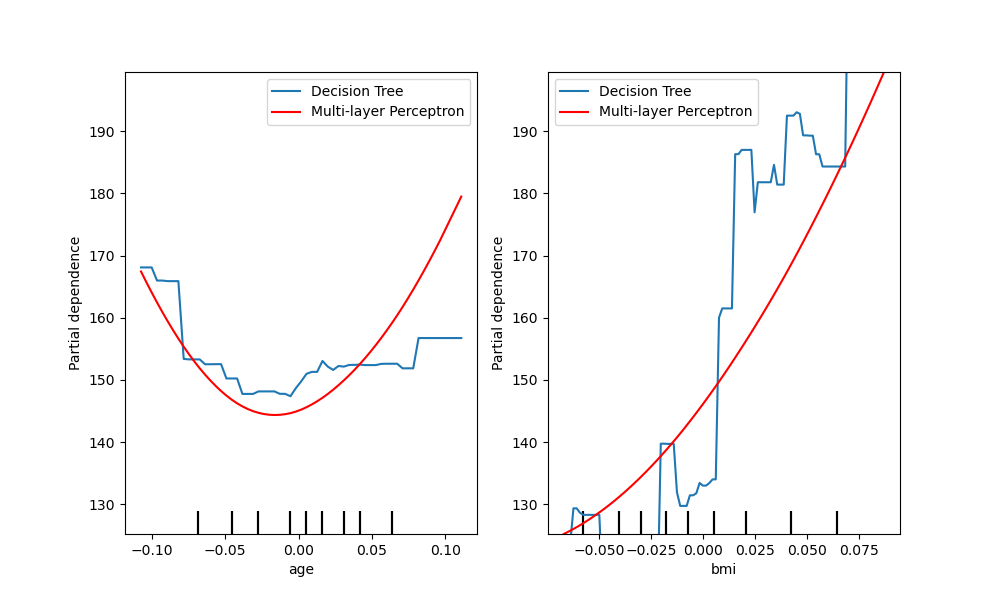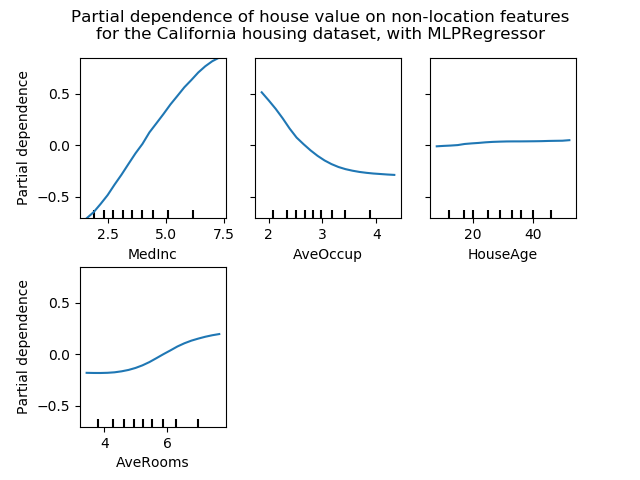# sklearn.inspection.plot_partial_dependence¶

sklearn.inspection.plot_partial_dependence(estimator, X, features, *, feature_names=None, target=None, response_method='auto', n_cols=3, grid_resolution=100, percentiles=(0.05, 0.95), method='auto', n_jobs=None, verbose=0, fig=None, line_kw=None, contour_kw=None, ax=None)

>>> from sklearn.inspection import plot_partial_dependence>>> from sklearn.datasets import make_friedman1>>> from sklearn.linear_model import LinearRegression>>> X, y = make_friedman1()>>> est = LinearRegression().fit(X, y)>>> disp1 = plot_partial_dependence(est, X)  >>> disp2 = plot_partial_dependence(est, X,...                                 ax=disp1.axes_)  

estimator BaseEstimator

X {array-like or dataframe} of shape (n_samples, n_features)
X用于为目标features(其中部分依赖将被评估)生成一个值网格，也用于在method为‘brute’时为补充特征生成值。
features list of {int, str, pair of int, pair of str}

feature_names array-like of shape (n_features,), dtype=str, default=None

target int, optional (default=None)
- 在多类设置中，指定应为其计算PDP的类。请注意，对于二进制分类，始终使用正类（索引为1）。
- 在多输出设置中，指定应为其计算PDP的任务。

response_method ‘auto’, ‘predict_proba’ or ‘decision_function’, optional (default=’auto’)

n_cols int, optional (default=3)

grid_resolution int, optional (default=100)

percentiles tuple of float, optional (default=(0.05, 0.95))

method str, optional (default=’auto’)

- “recursion”只支持一些基于树的估计（即 GradientBoostingClassifierGradientBoostingRegressorHistGradientBoostingClassifierHistGradientBoostingRegressorDecisionTreeRegressorRandomForestRegressor 但在速度方面更有效率。通过这种方法，分类的目标响应始终是决策函数，而不是预测的概率。

- 'brute' 任何估算器均支持，但计算量更大。
- 'auto'：'recursion'用于支持它的估计器，否则使用'brute'。

n_jobs int, optional (default=None)

verbose int, optional (default=0)
PD计算期间的输出详细程度。
fig Matplotlib figure object, optional (default=None)

line_kw dict, optional

contour_kw dict, optional

ax Matplotlib axes or array-like of Matplotlib axes, default=None
- 如果传入单个轴，则将其视为边界轴，并在这些边界内绘制部分依赖图网格。n_cols参数控制网格中的列数。
- 如果传入一个类数组的轴，则部分依赖图将直接绘制到这些轴中。
- 如果None，则创建图形和边界轴，并将其视为单轴情况。

display PartialDependenceDisplay

>>> from sklearn.datasets import make_friedman1>>> from sklearn.ensemble import GradientBoostingRegressor>>> X, y = make_friedman1()>>> clf = GradientBoostingRegressor(n_estimators=10).fit(X, y)>>> plot_partial_dependence(clf, X, [0, (0, 1)]) #doctest: +SKIP

## sklearn.inspection.plot_partial_dependence使用示例¶